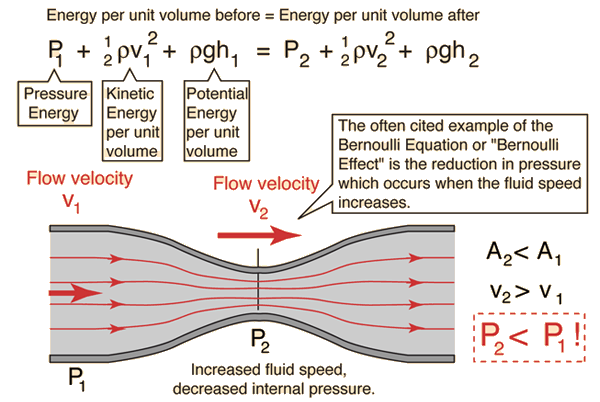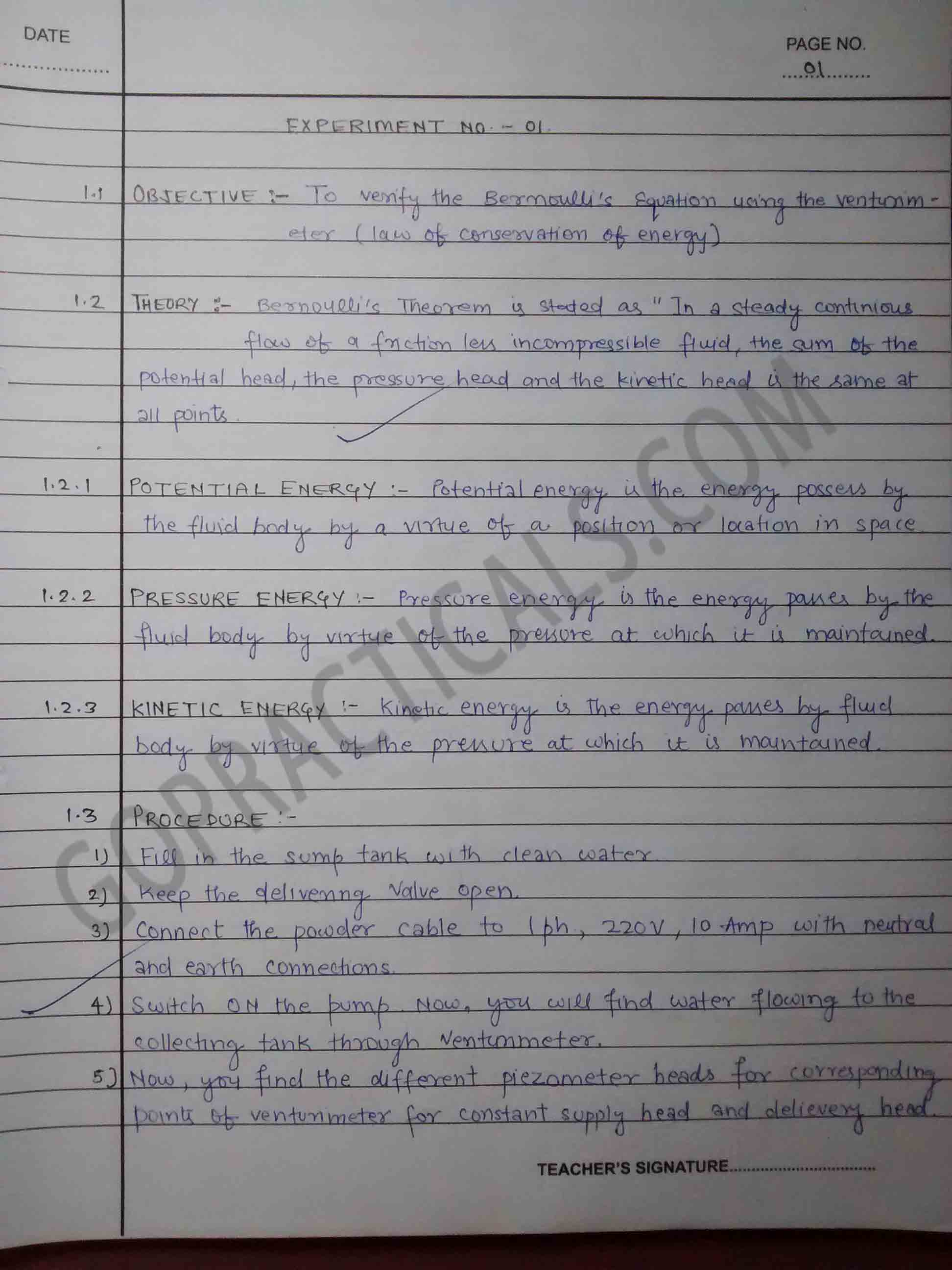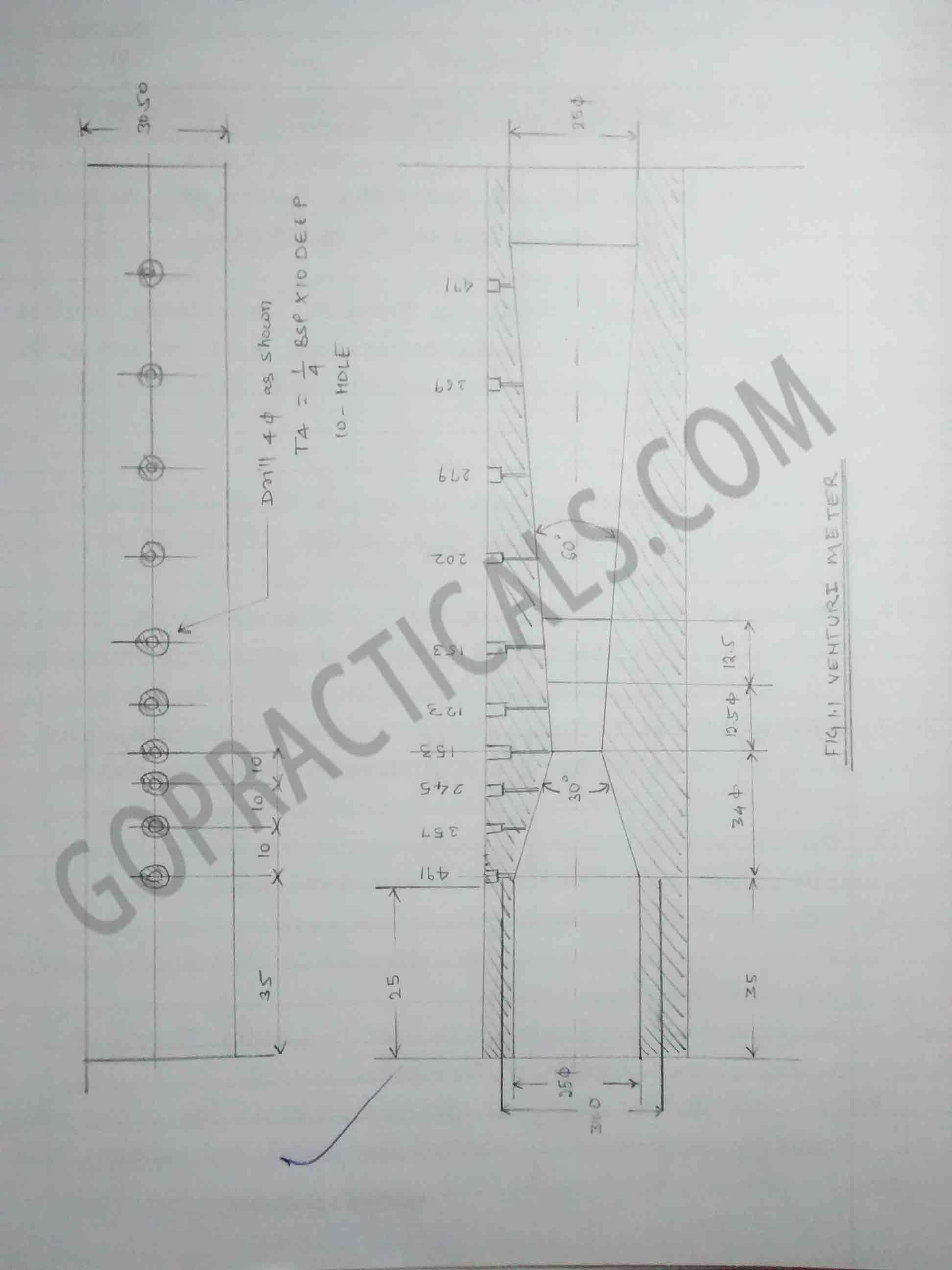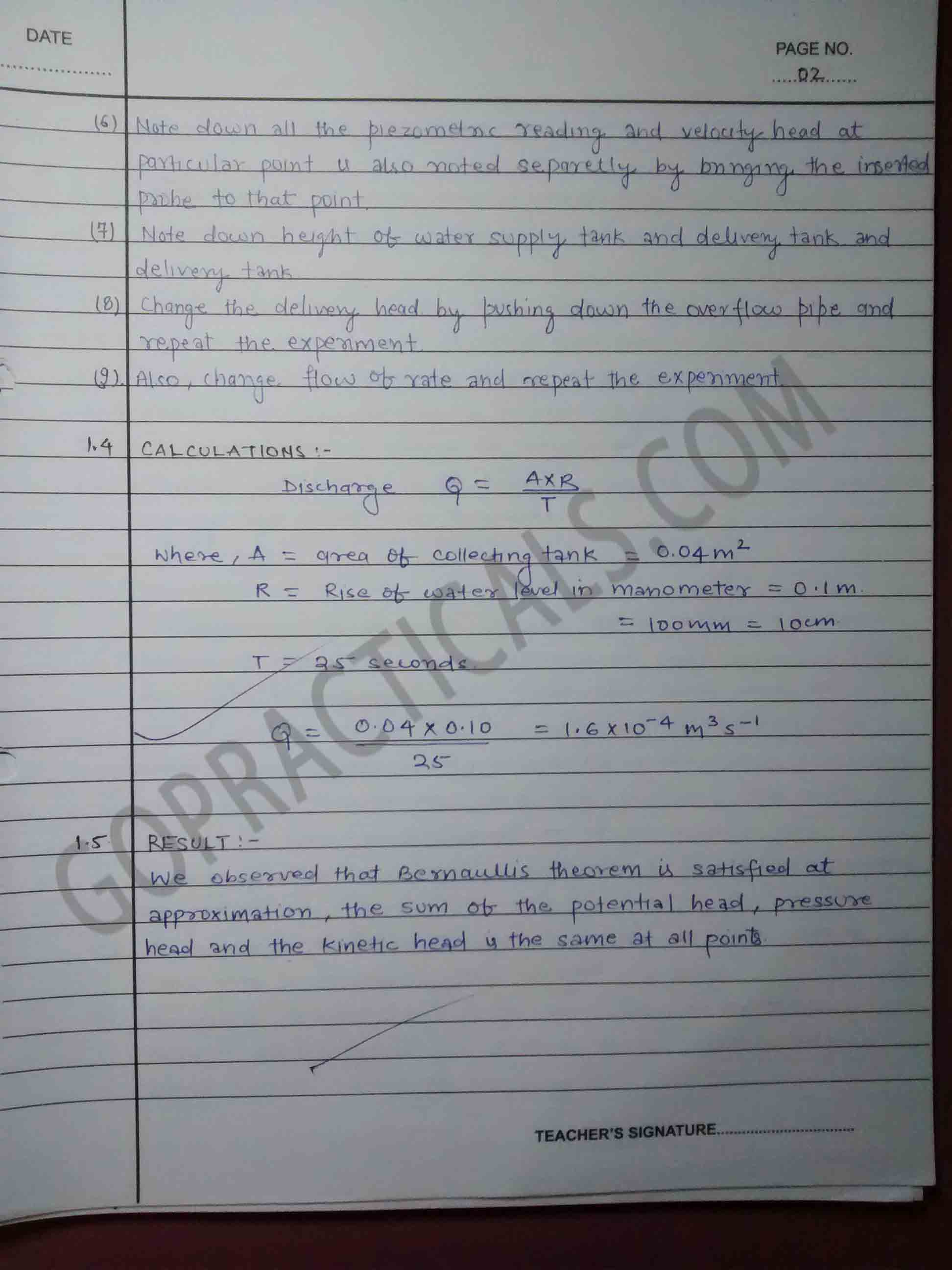The Bernoulli Equation can be considered to be a statement of the conservation of energy principle appropriate for flowing fluids. The qualitative behavior that is usually labeled with the term “Bernoulli effect” is the lowering of fluid pressure in regions where the flow velocity is increased. This lowering of pressure in a constriction of a flow path may seem counter intuitive, but seems less so when you consider pressure to be energy density. In the high velocity flow through the constriction, kinetic energy must increase at the expense of pressure energy.## Practical to verify Bernoulli’s equation using venturimeter## Watch this Video to know more about Bernoulli’s equation

Also Check Out – Consistency of Concrete by Slump Cone

Thanks for visiting us…

Verification of Bernoulli’s equation using Venturimeter – Fluid Practicals
Tagged on: